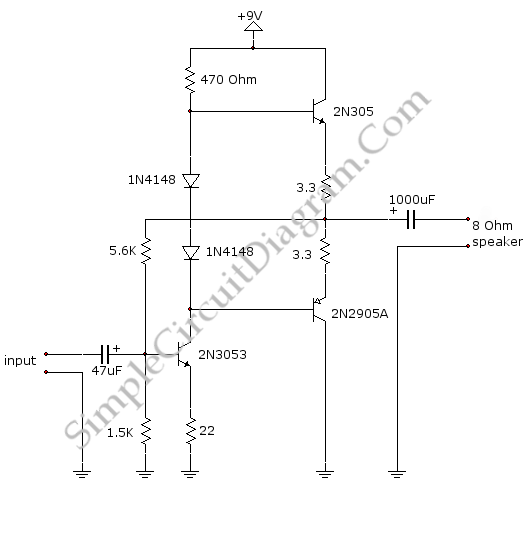# Small Discrete Audio Power Amplifier

This is a little audio amplifier, it is similar to the audio amplifier which is used in small transistor radio. This circuit draws about 30 milliamps from a 9 volt supply. This circuit consist of two stage. First stage is input stage (2N3053 transistor)  and the other is output stage (2N3053 and  2N2905 transistor pair). Here is the schematic diagram of the circuit :Supply voltage is divided equally across the two complimentary output transistors which are slightly biased in conduction by the diodes between the bases because the input stage is biased. To stabilize the bias current so it doesn’t change much with different transistors and diodes or with temperature. This circuit uses a 3.3 ohm resistor that assembled in series with the emitters of the output transistors. The voltage between the base and emitter decreased, because the bias current increased, so it is reduce the conduction. This circuit has voltage gain about 5 with an 8 ohm speaker attached and input impedance about 500 ohms.

In the output stage, the voltage swing on the speaker is about 2 volts without distorting. The power output of this circuit is in the 50 milliwatt range. To provide more power we must use the addition of heat sinks to the output transistors and a higher supply voltage.